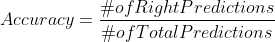1601250000

# The Ultimate Guide of Classification Metrics for Model Evaluation

Model evaluation is an essential part of machine learning. In dealing with classification problems, there are so many choices of metrics and sometimes it causes confusion. This article will first discuss and compare all the commonly used metrics for a binary classification problem, not only by their definitions, but also by the situations when some metrics are preferred over the others. Lastly, this article will discuss how to adjust the model in favor of certain metrics.

Common metrics for binary classification problems

Binary classification problems are a typical supervised machine learning problem with binary target values. We usually refer to the target values as positive class and negative class. When evaluating the performance of a model, the most common and straightforward metric to use is Accuracy. Accuracy is the number of right prediction divided by total prediction:#machine-learning #precision-recall #auc #hypothesis-testing #model-selection